﻿ 拉盖尔–高斯光束在小尺度流场内的气动光学效应 Aero-Optical Effect of Laguerre-Gaussian Vortex Beams through a Small-Scale Flow Field

Modern Physics
Vol.07 No.06(2017), Article ID:22886,9 pages
10.12677/MP.2017.76032

Aero-Optical Effect of Laguerre-Gaussian Vortex Beams through a Small-Scale Flow Field

Qianwen Jiang1, Yu Xin1*, Zhichao Zhou2, Qi Zhao1, Lingfei Xu2, Qibo Zhang1

1School of Electronic and Optical Engineering, Nanjing University of Science and Technology, Nanjing Jiangsu

2Shanghai Electro-Mechanical Engineering Institute, Shanghai

*通讯作者。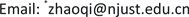Received: Nov. 9th, 2017; accepted: Nov. 24th, 2017; published: Nov. 30th, 2017ABSTRACT

To learn about the propagation characteristics of Laguerre-Gaussian (LG) vortex beams through the aero-optical environment formed by a micro-turret, the parabolic beam equations of complex amplitude were solved by second-order compact differences and Runge-Kutta integration was used for integral along the optical path. Strehl ratio (SR) and imaging displacement were calculated at different Mach numbers, different angles of attack, different altitudes and compared among Gaussian beam and LG beams with different topological charges. Simulations show that in the same flow field, the LG beam which has more topological charges, the phase of it has better stability and is always better than that of Gaussian beam, while the amplitude of it has worse stability, only when the radial mode index equals 0 and topological charge equals 1, the amplitude stability of LG beam is better than that of Gaussian beam.

Keywords:Aero-Optics, Propagation Characteristics, Numerical Simulation, Laguerre-Gaussian Vortex Beams, Small-Scale

1南京理工大学电子工程与光电技术学院，江苏 南京

2上海机电工程研究所，上海1. 引言

2. 理论分析

2.1. 气动模型

LG光束所经过的流场为一由微小凸起(以下简称凸起)造成的流场。凸起为一圆柱体加上半球体构成，置于一平面中心，即凸起底面圆心位置为(0,0)，如图1所示。截取凸起正上方2 × 2 × 1.73 mm3的流场作为光束要经过的小尺度流场。在LG光束传播1.73 mm后，可认为光束进入到折射率均匀的大气之中，一般而言，2 mm左右的传播距离已经能够使得光束表现出气动光学应。选用小尺度流场则是因为小尺度时流场的雷诺数较小，湍流激烈程度也较小，在流场仿真中更易形成稳定可收敛的流场。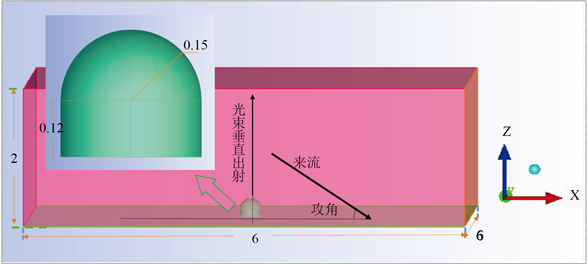Figure 1. Flow field parameters (in: mm)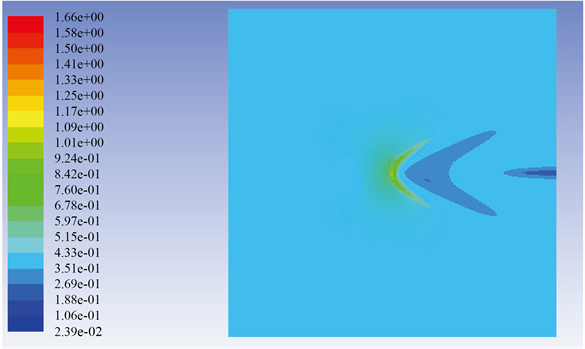Figure 2. Contour of density

2.2. 传输模型

${\nabla }^{2}U\left(r\right)+{k}^{2}{n}^{2}\left(r\right)U\left(r\right)=0$(1)

$f\left(z,A\right)\approx \frac{i}{2k}{\nabla }_{\perp }^{2}A+\frac{ik}{2}\left[{\left(\frac{n}{{n}_{r}}\right)}^{2}-1\right]A$(2)

$\Delta =\frac{\lambda }{2\text{π}}\mathrm{Re}\left\{-iIn\frac{{A}^{n+1}}{{A}_{r}^{n+1}}\right\}$(3)

$\stackrel{˜}{E}\left(x,y\right)=\frac{1}{i\lambda {z}_{0}}\mathrm{exp}\left[ik\left({z}_{0}+\frac{{x}^{2}+{y}^{2}}{2{z}_{0}}\right)\right]\underset{j=1}{\overset{\frac{2a}{\Delta {y}_{1}}}{\sum }}\underset{i=1}{\overset{\frac{2b}{\Delta {x}_{1}}}{\sum }}\mathrm{exp}\left[-ik\left(\frac{x}{{z}_{0}}{x}_{1}+\frac{y}{{z}_{0}}{y}_{1}\right)\right]{A}^{n+1}\left({x}_{1},{y}_{1}\right)\Delta {x}_{1}\Delta {y}_{1}$(4)

$\text{SR}=\mathrm{exp}\left[-{\left(k\cdot {\Delta }_{rms}\right)}^{2}\right]$ , (5)

$\Delta \stackrel{¯}{r}=\stackrel{¯}{r}-{\stackrel{¯}{r}}_{0}$ , (6)

3. 振幅和相位稳定性

$A\left(x,y,z\right)=\frac{\mathrm{exp}\left[-{a}_{0}k\frac{{x}^{2}+{y}^{2}}{2\left(1+i{a}_{0}z\right)}\right]}{1+i{a}_{0}z}$ , (7)

$\begin{array}{c}A\left(x,y,z,p,l\right)=\sqrt{\frac{2p!}{\text{π}\left(|l|+p\right)!}}\cdot \frac{1}{\omega \left(z\right)}\cdot \mathrm{exp}\left[i\left(2p+|l|+1\right)\phi \left(z\right)\right]\cdot {\left[\frac{\sqrt{2\left({x}^{2}+{y}^{2}\right)}}{\omega \left(z\right)}\right]}^{|l|}\\ \text{\hspace{0.17em}}\text{\hspace{0.17em}}\cdot {L}_{p}^{|l|}\left[\frac{2\left({x}^{2}+{y}^{2}\right)}{{\omega }^{2}\left(z\right)}\right]\cdot \mathrm{exp}\left[-ik\frac{{x}^{2}+{y}^{2}}{2q\left(z\right)}+il\mathrm{arctan}\left(\frac{y}{x}\right)\right]\end{array}$(8)

3.1. 光束的相位稳定性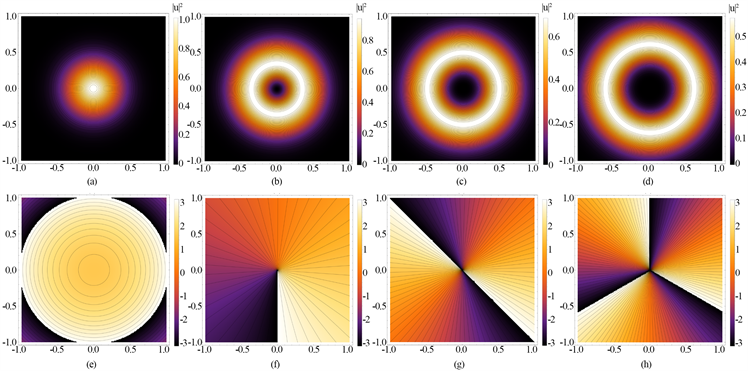Figure 3. (a)~(d) Intensity, (e)~(h) Phase, (a, e) Gaussian beam, (b, f) L1, (c, g) L2, (d, h) L3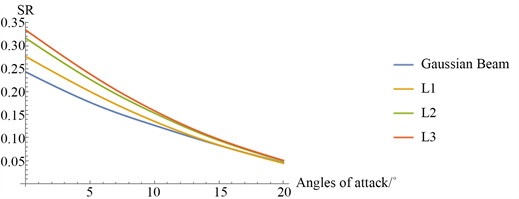Figure 4. SR at different angles of attack

3.2. 光束的振幅稳定性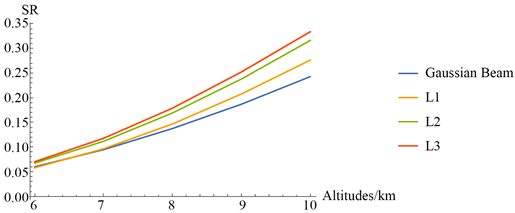Figure 5. SR at different altitudes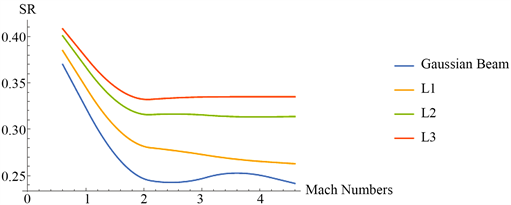Figure 6. SR at different Mach Numbers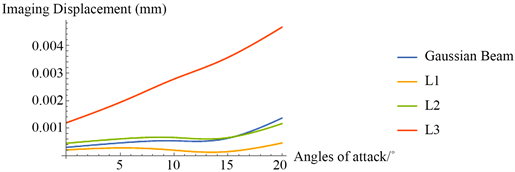Figure 7. Imaging displacements at different angles of attack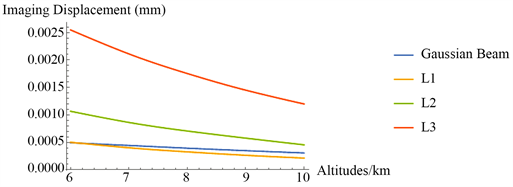Figure 8. Imaging displacements at different altitudes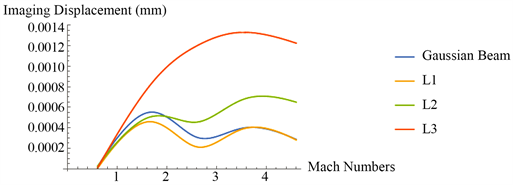Figure 9. Imaging displacement at different Mach numbers

4. 结论

Aero-Optical Effect of Laguerre-Gaussian Vortex Beams through a Small-Scale Flow Field[J]. 现代物理, 2017, 07(06): 273-281. http://dx.doi.org/10.12677/MP.2017.76032

1. 1. Coullet, P., Gil, L. and Rocca, F. (1989) Optical Vortices. Optical Communications, 73, 403-408. https://doi.org/10.1016/0030-4018(89)90180-6

2. 2. Baumann, S.M., Kalb, D.M., MacMillan, L.H., et al. (2009) Propagation Dynamics of Optical Vortices Due to Gouy Phase. Optics Express, 17, 9818-9827. https://doi.org/10.1364/OE.17.009818

3. 3. Lochab, P., Senthilkumaran, P. and Khare, K. (2016) Near-Core Structure of a Propagating Optical Vortex. Journal of the Optical Society of America A, 33, 2485-2490. https://doi.org/10.1364/JOSAA.33.002485

4. 4. Zhang, Y., Djordjevic, I.B. and Gao, X. (2012) On the Quantum-Channel Ca-pacity for Orbital Angular Momentum-Based Free-Space Optical Communications. Optics Letters, 37, 3267-3269. https://doi.org/10.1364/OL.37.003267

5. 5. Tang, M. and Zhao, D. (2015) Propagation of Multi-Gaussian Schell-Model Vortex Beams in Isotropic Random Media. Optics Express, 23, 32766. https://doi.org/10.1364/OE.23.032766

6. 6. Aksenov, V.P., Kolosov, V.V. and Pogutsa, C.E. (2014) Random Wandering of Laser Beams with Orbital Angular Momentum during Propagation through Atmospheric Turbulent. Applied Optics, 53, 3607-3614. https://doi.org/10.1364/AO.53.003607

7. 7. Morgan, P., White, M. and Visbal, M. (2011) Simulation of Aero-Optics for Flow Over a Flat-Window Hemispherical Turret. Aiaa Fluid Dynamics Conference and Exhibit, 453-458. https://doi.org/10.2514/6.2011-3264

8. 8. Gordeyev, S., Jumper, E. and Hayden, T. (2013) Aero-Optics of Supersonic Boundary Layers. Aiaa Journal, 50, 682- 690. https://doi.org/10.2514/1.J051266

9. 9. Gao, Q., Jiang, Z., Yi, S., et al. (2013) Statistical Characteristics of the Tilts of the Aero-Optical Aberration Caused by the Supersonic Turbulent Boundary Layer. Optics Letters, 38, 751-753. https://doi.org/10.1364/OL.38.000751

10. 10. John, P. Siegenthaler, S.G. and Jumper, E. (2005) Shear Layers and Aper-ture Effects for Aero-Optics. 36th AIAA Plasmadynamics and Lasers Conference.

11. 11. White, M.D. (2010) High-Order Parabolic Beam Approximation for Aero-Optics. Journal of Computational Physics, 229, 5465-5485. https://doi.org/10.1016/j.jcp.2010.03.046

12. 12. Mahajan, V.N. (1983) StrehlRatio for Aberration in Terms of Their Aberration Variance. Journal of the Optical Society of America A, 73, 860-861. https://doi.org/10.1364/JOSA.73.000860

13. 13. Levy, U., Derevyanko, S. and Silberberg, Y. (2016) Light Modes of Free Space. Progress in Optics, 61, 237-281. https://doi.org/10.1016/bs.po.2015.10.001